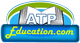ATP Education

Page 1 of 2

1. Rational Numbers

Exercise 1.1

Exercise 1.1

Q1. Using appropriate properties find;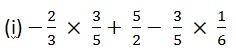Solution:Using BODMAS first we solve for multiply here;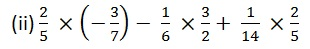Solution:Using BODMAS first we solve for multiply here;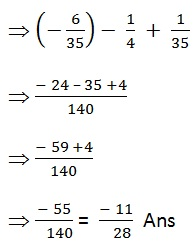Q2. Write the additive inverse of each of the following.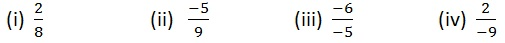Solution:Q3. Verify that – (- x) = x for;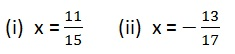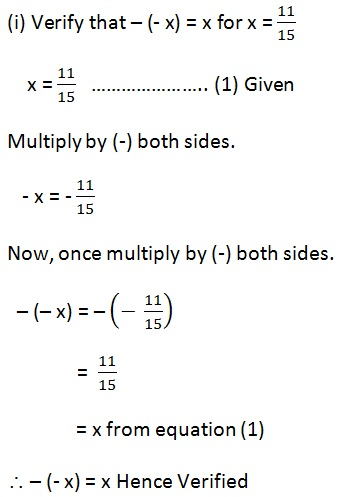ATP Education
www.atpeducation.com ATP Education www.atpeducation.com

ATP Education

Page 1 of 2

See other sub-topics of this chapter: